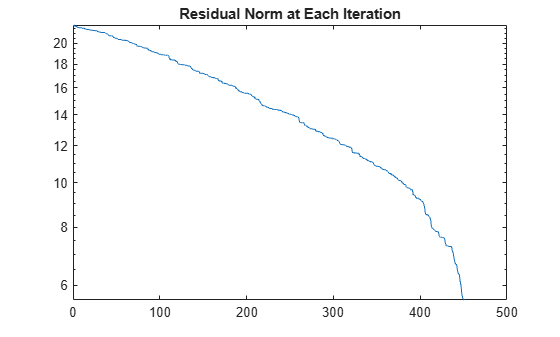equilibrate

Matrix scaling for improved conditioning

Description

example

[P,R,C] = equilibrate(A) permutes and rescales matrix A such that the new matrix B = R*P*A*C has a diagonal with entries of magnitude 1, and its off-diagonal entries are not greater than 1 in magnitude.

Examples

collapse all

Equilibrate a matrix with a large condition number to improve the efficiency and stability of a linear system solution with the iterative solver gmres.

Load the west0479 matrix, which is a real-valued 479-by-479 sparse matrix. Use condest to calculate the estimated condition number of the matrix.

A = west0479;
c1 = condest(A)
c1 = 1.4244e+12

Try to solve the linear system $\mathrm{Ax}=\mathit{b}$ using gmres with 450 iterations and a tolerance of 1e-11. Specify five outputs so that gmres returns the residual norms of the solution at each iteration (using ~ to suppress unneeded outputs). Plot the residual norms in a semilog plot. The plot shows that gmres is not able to achieve a reasonable residual norm, and so the calculated solution for $\mathit{x}$ is not reliable.

b = ones(size(A,1),1);
tol = 1e-11;
maxit = 450;
[x,flx,~,~,rvx] = gmres(A,b,[],tol,maxit);
semilogy(rvx)
title('Residual Norm at Each Iteration')Use equilibrate to permute and rescale A. Create a new matrix B = R*P*A*C, which has a better condition number and diagonal entries of only 1 and -1.

[P,R,C] = equilibrate(A);
B = R*P*A*C;
c2 = condest(B)
c2 = 5.1036e+04

Using the outputs returned by equilibrate, you can reformulate the problem $\mathrm{Ax}=\mathit{b}$ into $\mathrm{By}=\mathit{d}$, where $\mathit{B}=\mathrm{RPAC}$ and $\mathit{d}=\mathrm{RPb}$. In this form you can recover the solution to the original system with $\mathit{x}=\mathrm{Cy}$.

Use gmres to solve $\mathrm{By}=\mathit{d}$ for $\mathit{y}$, and then replot the residual norms at each iteration. The plot shows that equilibrating the matrix improves the stability of the problem, with gmres converging to the desired tolerance of 1e-11 in fewer than 200 iterations.

d = R*P*b;
[y,fly,~,~,rvy] = gmres(B,d,[],tol,maxit);
hold on
semilogy(rvy)
legend('Original', 'Equilibrated', 'Location', 'southeast')
title('Relative Residual Norms (No Preconditioner)')
hold offImprove Solution with Preconditioner

After you obtain the matrix B, you can improve the stability of the problem even further by calculating a preconditioner for use with gmres. The numerical properties of B are better than those of the original matrix A, so you should use the equilibrated matrix to calculate the preconditioner.

Calculate two different preconditioners with ilu, and use these as inputs to gmres to solve the problem again. Plot the residual norms at each iteration on the same plot as the equilibrated norms for comparison. The plot shows that calculating preconditioners with the equilibrated matrix greatly increases the stability of the problem, with gmres achieving the desired tolerance in fewer than 30 iterations.

semilogy(rvy)
hold on

[L1,U1] = ilu(B,struct('type','ilutp','droptol',1e-1,'thresh',0));
[yp1,flyp1,~,~,rvyp1] = gmres(B,d,[],tol,maxit,L1,U1);
semilogy(rvyp1)

[L2,U2] = ilu(B,struct('type','ilutp','droptol',1e-2,'thresh',0));
[yp2,flyp2,~,~,rvyp2] = gmres(B,d,[],tol,maxit,L2,U2);
semilogy(rvyp2)

legend('No preconditioner', 'ILUTP(1e-1)', 'ILUTP(1e-2)')
title('Relative Residual Norms with ILU Preconditioner (Equilibrated)')
hold offInput Arguments

collapse all

Input matrix, specified as a square matrix. A can be dense or sparse, but must be structurally nonsingular, as determined by sprank.

When A is sparse, the outputs P, R, and C are also sparse.

Data Types: single | double
Complex Number Support: Yes

Output Arguments

collapse all

Permutation matrix, returned as a matrix. P*A is the permutation of A that maximizes the absolute value of the product of its diagonal elements.

Row scaling, returned as a diagonal matrix. The diagonal entries in R and C are real and positive.

Column scaling, returned as a diagonal matrix. The diagonal entries in R and C are real and positive.

 Duff, I. S., and J. Koster. “On Algorithms For Permuting Large Entries to the Diagonal of a Sparse Matrix.” SIAM Journal on Matrix Analysis and Applications 22, no. 4 (January 2001): 973–96.